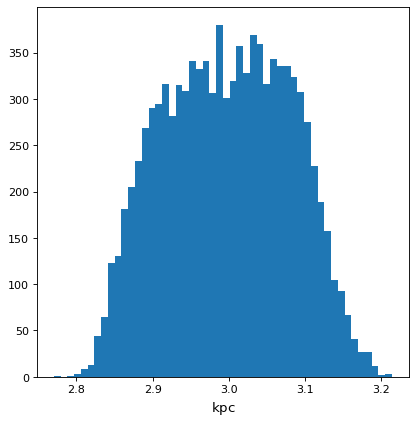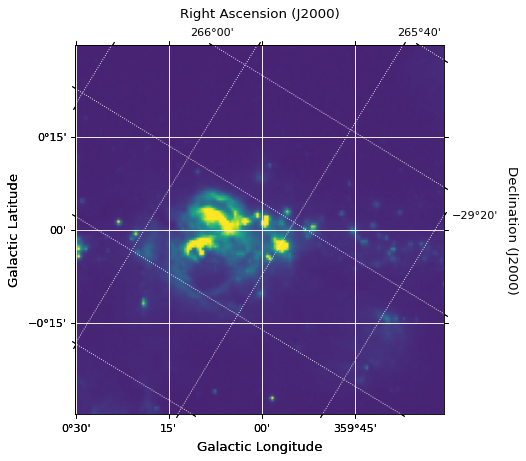# Making plots with world coordinates (WCSAxes)¶

WCSAxes is a framework for making plots of Astronomical data in Matplotlib. It was previously distributed as a standalone package, but is now included in astropy.visualization.

## Getting started¶

The following is a very simple example of plotting an image with the WCSAxes package:

import matplotlib.pyplot as plt

from astropy.wcs import WCS
from astropy.io import fits
from astropy.utils.data import get_pkg_data_filename

filename = get_pkg_data_filename('galactic_center/gc_msx_e.fits')

hdu = fits.open(filename)
wcs = WCS(hdu.header)

plt.subplot(projection=wcs)
plt.imshow(hdu.data, vmin=-2.e-5, vmax=2.e-4, origin='lower')
plt.grid(color='white', ls='solid')
plt.xlabel('Galactic Longitude')
plt.ylabel('Galactic Latitude')


(png, svg, pdf)This example uses the matplotlib.pyplot interface to Matplotlib, but WCSAxes can be used with any of the other ways of using Matplotlib (some examples of which are given in Initializing axes with world coordinates). For example, using the partially object-oriented interface, you can do:

ax = plt.subplot(projection=wcs)
ax.imshow(hdu.data, vmin=-2.e-5, vmax=2.e-4, origin='lower')
ax.grid(color='white', ls='solid')
ax.set_xlabel('Galactic Longitude')
ax.set_ylabel('Galactic Latitude')


However, the axes object is needed to access some of the more advanced functionality of WCSAxes. An example of this usage is:

ax = plt.subplot(projection=wcs)

ax.imshow(hdu.data, vmin=-2.e-5, vmax=2.e-4, origin='lower')

ax.coords.grid(True, color='white', ls='solid')
ax.coords.set_axislabel('Galactic Longitude')
ax.coords.set_axislabel('Galactic Latitude')

overlay = ax.get_coords_overlay('fk5')
overlay.grid(color='white', ls='dotted')
overlay.set_axislabel('Right Ascension (J2000)')
overlay.set_axislabel('Declination (J2000)')


(png, svg, pdf)In the rest of this documentation we will assume that you have kept a reference to the axes object, which we will refer to as ax. However, we also note when something can be done directly with the pyplot interface.

WCSAxes supports a number of advanced plotting options, including the ability to control which axes to show labels on for which coordinates, overlaying contours from data with different coordinate systems, overlaying grids for different coordinate systems, dealing with plotting slices from data with more than two dimensions, and defining custom (non-rectangular) frames.

## Reference/API¶

### astropy.visualization.wcsaxes Package¶

#### Classes¶

 Conf Configuration parameters for astropy.visualization.wcsaxes. CoordinateHelper([parent_axes, parent_map, …]) Helper class to control one of the coordinates in the WCSAxes. CoordinatesMap(axes[, wcs, transform, …]) A container for coordinate helpers that represents a coordinate system. SphericalCircle(center, radius[, …]) Create a patch representing a spherical circle - that is, a circle that is formed of all the points that are within a certain angle of the central coordinates on a sphere. WCSAxes(fig, rect[, wcs, transform, …]) The main axes class that can be used to show world coordinates from a WCS. WCSAxesSubplot(fig, *args, **kwargs) A subclass class for WCSAxes

### astropy.visualization.wcsaxes.frame Module¶

#### Classes¶

 Spine(parent_axes, transform) A single side of an axes. BaseFrame(parent_axes, transform[, path]) Base class for frames, which are collections of Spine instances. RectangularFrame(parent_axes, transform[, path]) A classic rectangular frame. EllipticalFrame(parent_axes, transform[, path]) An elliptical frame.